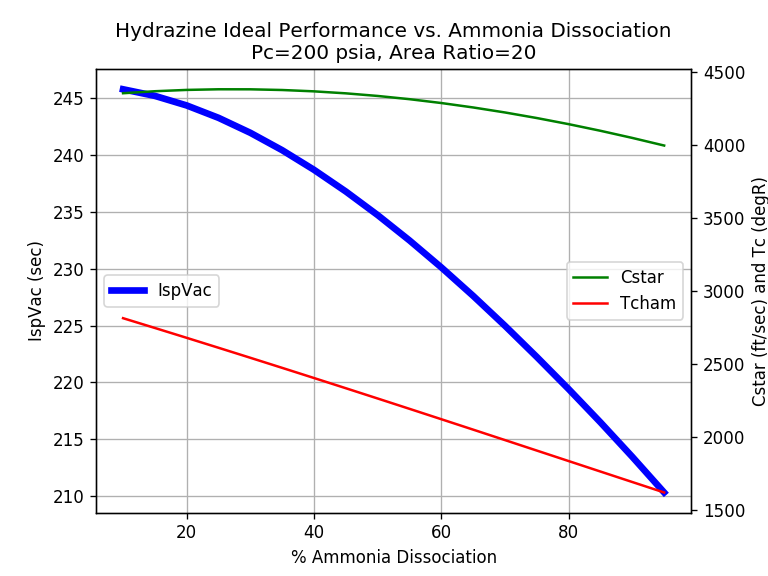# Hydrazine Monopropellant¶

## Chemical Reactions¶

When decomposing Hydrazine (N2H4) as a monopropellant, there are two successive reactions to consider:

```3 N2H4 --> 4 NH3 +   N2 (highly exothermic)
4 NH3  --> 2 N2  + 6 H2 (endothermic)
```

The first reaction is highly exothermic and goes to completion. It creates ammonia (NH3) and nitrogen gas (N2).

The second reaction (ammonia dissociation), however, can be controlled by the design of a catalyst bed. The more ammonia dissociation there is, the cooler the resulting combustion products and the lower the Isp of the monopropellant engine.

## CEA Modification¶

In RocketCEA the CEA FORTRAN code has been modified to include “Undissociated Ammonia (UA)” as an exhaust product. When decomposing hydrazine with only partial ammonia dissociation, the CEA input includes an “omit NH3” statement to prevent normal equilibrium NH3 calculations.

Partial ammonia dissociation is implemented in RocketCEA with the propName “HYDnn”, where “nn” is the mass percent of ammonia that dissociates. For example, 30, 40 and 50 percent dissociation would be “HYD30”, “HYD40” and “HYD50”.

This can be demonstrated with the following RocketCEA script that shows the difference between HYD40 (40% ammonia dissociation) and N2H4 (equilibrium CEA logic)

```from rocketcea.cea_obj import CEA_Obj

C40 =  CEA_Obj( propName='HYD40')
C100 = CEA_Obj( propName='N2H4')

I40,  C40,  T40 =   C40.get_IvacCstrTc(Pc=200.0, eps=20.0)
I100, C100, T100 = C100.get_IvacCstrTc(Pc=200.0, eps=20.0)

print('       Isp     Cstar     Tc')
print('      (sec)   (ft/sec)  (degR)')
print('40%%    %5.1f   %6.1f   %6.1f'%(I40, C40, T40) )
print('100%%   %5.1f   %6.1f   %6.1f'%(I100, C100, T100) )
```
```       Isp     Cstar     Tc
(sec)   (ft/sec)  (degR)
40%    238.7   4364.4   2404.1
100%   222.0   3995.1   1581.8
```

Note that high levels of ammonia dissociation can be beneficial in some applications like gas generators where lower combustion temperature is desirable. Typical ranges of ammonia dissociation for hydrazine monopropellant applications are:

```%Dissociation          Application
30% - 50%          High Performance Thrusters
50% - 70%          ACS Thrusters
60% - 80%          Gas Generators
```

## Performance Plot¶

A plot of Monopropellant Hydrazine Performance can be created with the following script.

```from rocketcea.cea_obj import CEA_Obj
import matplotlib.pyplot as plt

Pc = 200.0
eps = 20.0

xL = []    # save data to lists
ispL = []
cstarL = []
tcL = []

for x in range(10, 100, 5): # look at amm_dissociation from 5% to 95%
propName = 'HYD%g'%x
ispObj = CEA_Obj(propName=propName)

xL.append( x ) # save percent amm_dissociation

IspVac, Cstar, Tcomb = ispObj.get_IvacCstrTc( Pc=Pc, eps=eps)
ispL.append( IspVac ) # save IspVac
cstarL.append( Cstar )# save Cstar
tcL.append( Tcomb )   # save Tcomb

fig, ax1 = plt.subplots()
ax1.plot(xL, ispL, 'b-', label='IspVac', linewidth=4)

plt.grid(True)
plt.title( 'Hydrazine Ideal Performance vs. Ammonia Dissociation\nPc=%g psia, Area Ratio=%g'%(Pc, eps) )
ax1.set_xlabel( '% Ammonia Dissociation' )
ax1.set_ylabel( 'IspVac (sec)' )

ax2 = ax1.twinx()
ax2.set_ylabel('Cstar (ft/sec) and Tc (degR)')
ax2.plot(xL, cstarL, 'g-', label='Cstar')
ax2.plot(xL, tcL,    'r-', label='Tcham')

ax1.legend(loc='center left')
ax2.legend(loc='center right')

plt.savefig('amm_dissociation.png', dpi=120)
plt.show()
```Click Image to View Fill Size